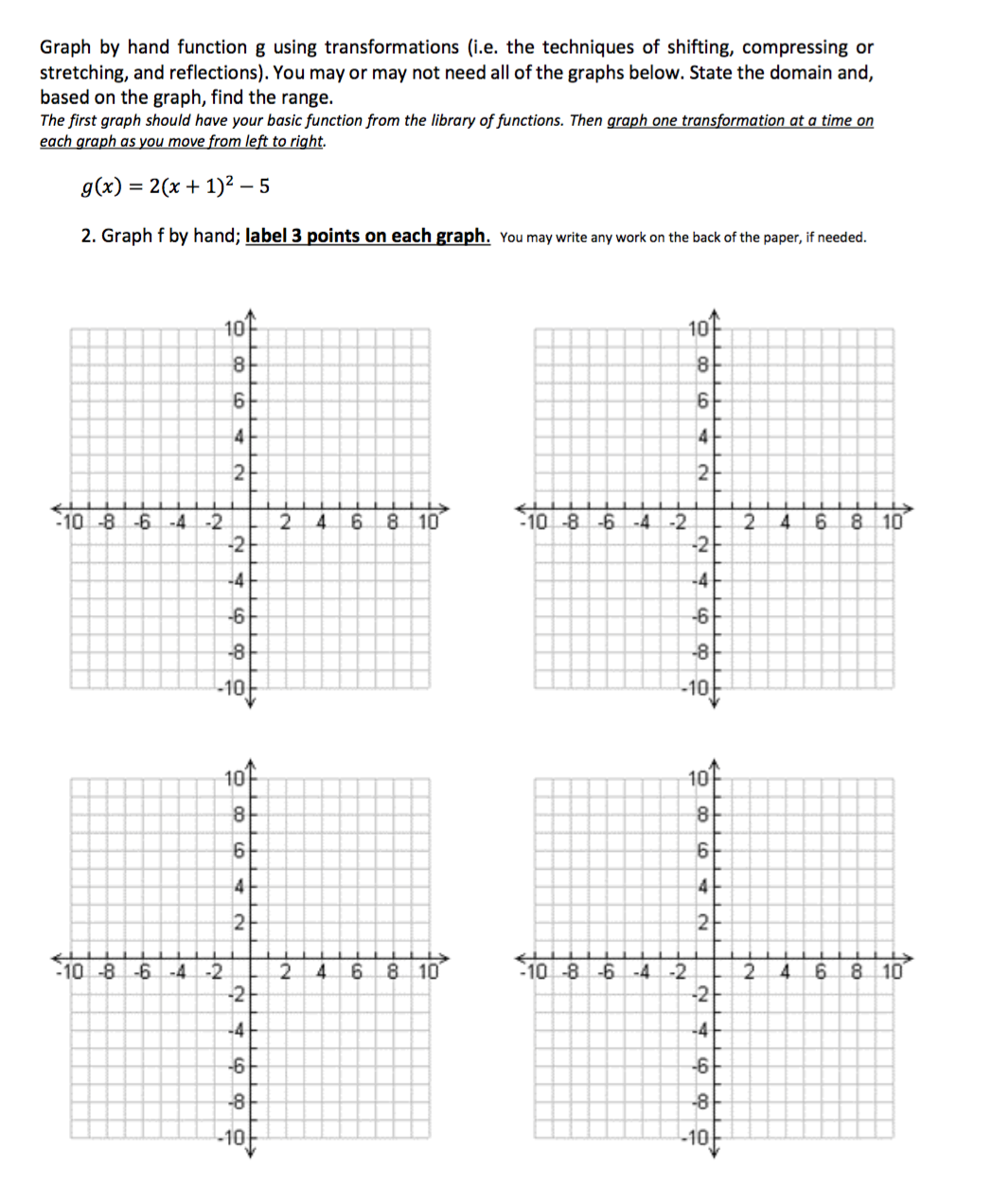Graph by hand function g using transformations (i.e. the techniques of shifting, compressing orstretching, and reflections). You may or may not need all of the graphs below. State the domain and,based on the graph, find the range.The first graph should have your basic function from the library of functions. Then graph one transformation at a time ong(x) 2(x + 1)2- 52. Graph f by hand; label 3 points on each graph. You may write any work on the back of the paper, if needed1010810 -8 6 -4-22 46810102 468 101010101010-86 -4-22 46810O-102 46 81O1010

Questionhelp_outlineImage TranscriptioncloseGraph by hand function g using transformations (i.e. the techniques of shifting, compressing or stretching, and reflections). You may or may not need all of the graphs below. State the domain and, based on the graph, find the range. The first graph should have your basic function from the library of functions. Then graph one transformation at a time on g(x) 2(x + 1)2- 5 2. Graph f by hand; label 3 points on each graph. You may write any work on the back of the paper, if needed 10 10 8 10 -8 6 -4-2 2 46810 10 2 468 10 10 10 10 10 10-86 -4-2 2 46810O -10 2 46 81O 10 10 fullscreen
Step 1

Given:

The function is,

Step 2

To sketch the graph of the function g(x), using transformation as shown in the following  graphs.

Consider the function  y = x² .

Substitute the values x = 0,  x = 2 and x = –2 in the above function.

Then the corresponding y  values are y = 0,  y = 4 and y = 4.

That is, the points required to draw the graph y = x² are (0,0), (–2,4) and (2,4).

Sketch the graph y = x² as shown in the below figure.

Step 3

Translate the function y = x² by the left of 1 unit.

Then the translation  function is  y = (x+1)² .

Substitute the values x = –1,  x = –4 and x = 2 in the above function.

Then the corresponding y  values are y = 0,  y = 9 and y = 9.

...

Want to see the full answer?

See Solution

Want to see this answer and more?

Our solutions are written by experts, many with advanced degrees, and available 24/7

See Solution
Tagged in

Other